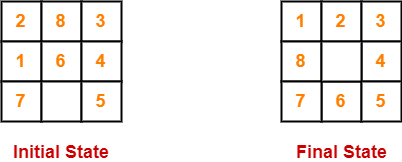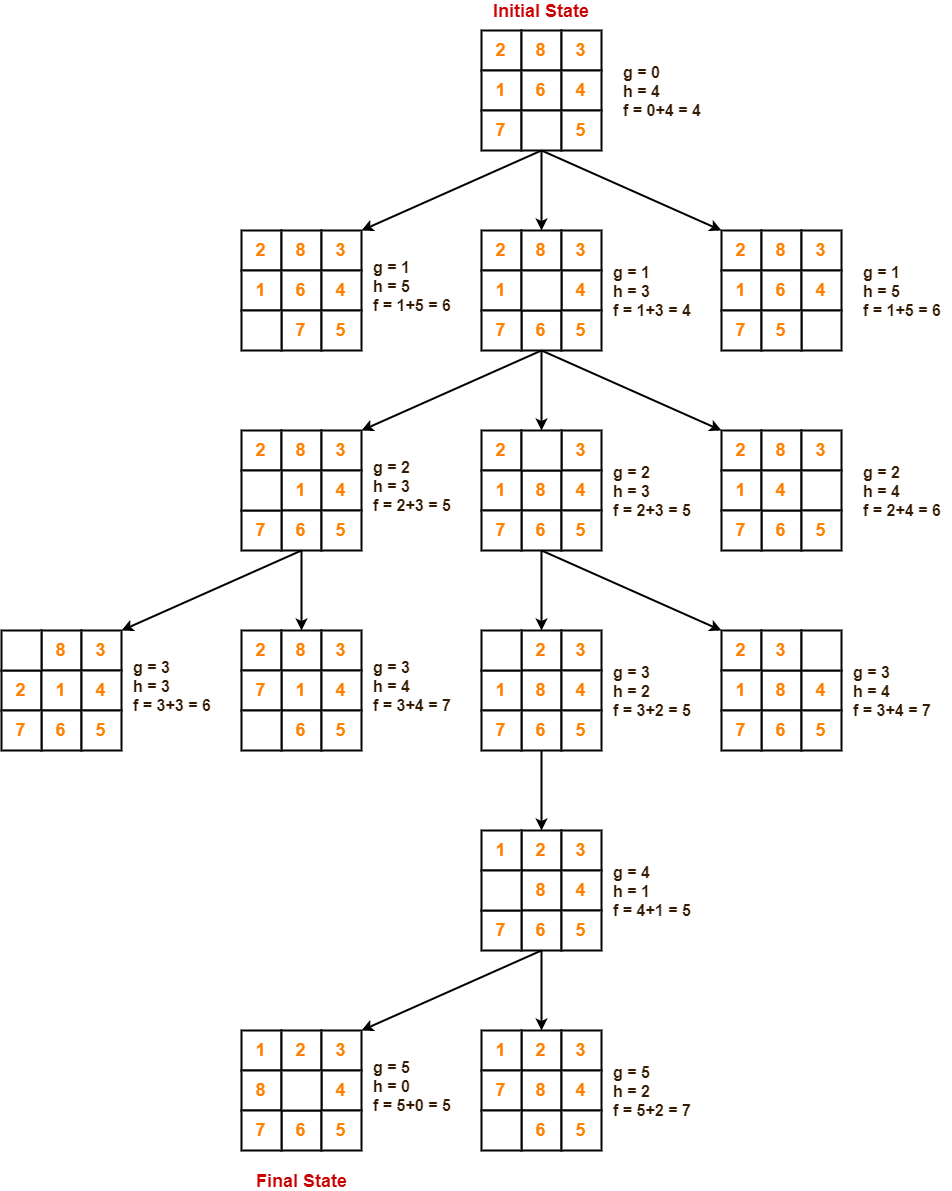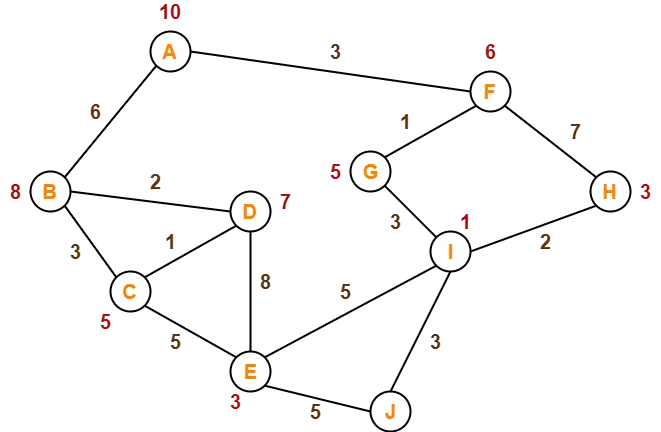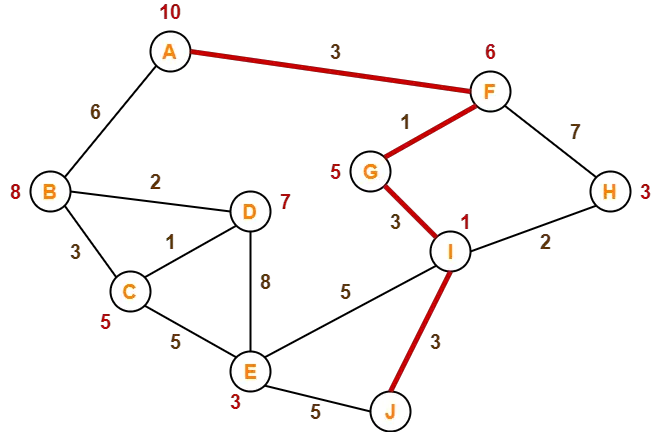# A* Algorithm | A* Algorithm Example in AI

## A* Algorithm-

• A* Algorithm is one of the best and popular techniques used for path finding and graph traversals.
• A lot of games and web-based maps use this algorithm for finding the shortest path efficiently.
• It is essentially a best first search algorithm.

## Working-

A* Algorithm works as-

• It maintains a tree of paths originating at the start node.
• It extends those paths one edge at a time.
• It continues until its termination criterion is satisfied.

A* Algorithm extends the path that minimizes the following function-

f(n) = g(n) + h(n)

Here,

• ‘n’ is the last node on the path
• g(n) is the cost of the path from start node to node ‘n’
• h(n) is a heuristic function that estimates cost of the cheapest path from node ‘n’ to the goal node

## Algorithm-

• The implementation of A* Algorithm involves maintaining two lists- OPEN and CLOSED.
• OPEN contains those nodes that have been evaluated by the heuristic function but have not been expanded into successors yet.
• CLOSED contains those nodes that have already been visited.

The algorithm is as follows-

### Step-01:

• Define a list OPEN.
• Initially, OPEN consists solely of a single node, the start node S.

### Step-02:

If the list is empty, return failure and exit.

### Step-03:

• Remove node n with the smallest value of f(n) from OPEN and move it to list CLOSED.
• If node n is a goal state, return success and exit.

Expand node n.

### Step-05:

• If any successor to n is the goal node, return success and the solution by tracing the path from goal node to S.
• Otherwise, go to Step-06.

### Step-06:

For each successor node,

• Apply the evaluation function f to the node.
• If the node has not been in either list, add it to OPEN.

### Step-07:

Go back to Step-02.

## Problem-01:

Given an initial state of a 8-puzzle problem and final state to be reached-Find the most cost-effective path to reach the final state from initial state using A* Algorithm.

Consider g(n) = Depth of node and h(n) = Number of misplaced tiles.

## Solution-

• A* Algorithm maintains a tree of paths originating at the initial state.
• It extends those paths one edge at a time.
• It continues until final state is reached.## Problem-02:

Consider the following graph-The numbers written on edges represent the distance between the nodes.

The numbers written on nodes represent the heuristic value.

Find the most cost-effective path to reach from start state A to final state J using A* Algorithm.

## Solution-

### Step-01:

• Node B and Node F can be reached from node A.

A* Algorithm calculates f(B) and f(F).

• f(B) = 6 + 8 = 14
• f(F) = 3 + 6 = 9

Since f(F) < f(B), so it decides to go to node F.

Path- A → F

### Step-02:

Node G and Node H can be reached from node F.

A* Algorithm calculates f(G) and f(H).

• f(G) = (3+1) + 5 = 9
• f(H) = (3+7) + 3 = 13

Since f(G) < f(H), so it decides to go to node G.

Path- A → F → G

### Step-03:

Node I can be reached from node G.

A* Algorithm calculates f(I).

f(I) = (3+1+3) + 1 = 8

It decides to go to node I.

Path- A → F → G → I

### Step-04:

Node E, Node H and Node J can be reached from node I.

A* Algorithm calculates f(E), f(H) and f(J).

• f(E) = (3+1+3+5) + 3 = 15
• f(H) = (3+1+3+2) + 3 = 12
• f(J) = (3+1+3+3) + 0 = 10

Since f(J) is least, so it decides to go to node J.

Path- A → F → G → I → J

This is the required shortest path from node A to node J.## Important Note-

It is important to note that-

• A* Algorithm is one of the best path finding algorithms.
• But it does not produce the shortest path always.
• This is because it heavily depends on heuristics.

To gain better understanding about A* Search Algorithm,

Watch this Video Lecture

Get more notes and other study material of Artificial Intelligence.

Watch video lectures by visiting our YouTube channel LearnVidFun.

SummaryArticle Name
A* Algorithm | A* Algorithm Example in AI
Description
A* Algorithm in Artificial Intelligence is a popular path finding technique. A* Algorithm Working & Pseudo Code. A* Algorithm Examples in AI. A* Algorithm on 8 Puzzle Problem is discussed.
Author
Publisher Name
Gate Vidyalay
Publisher Logo